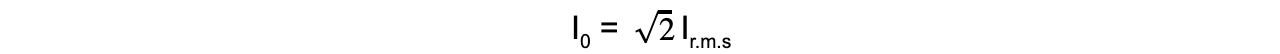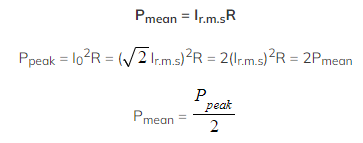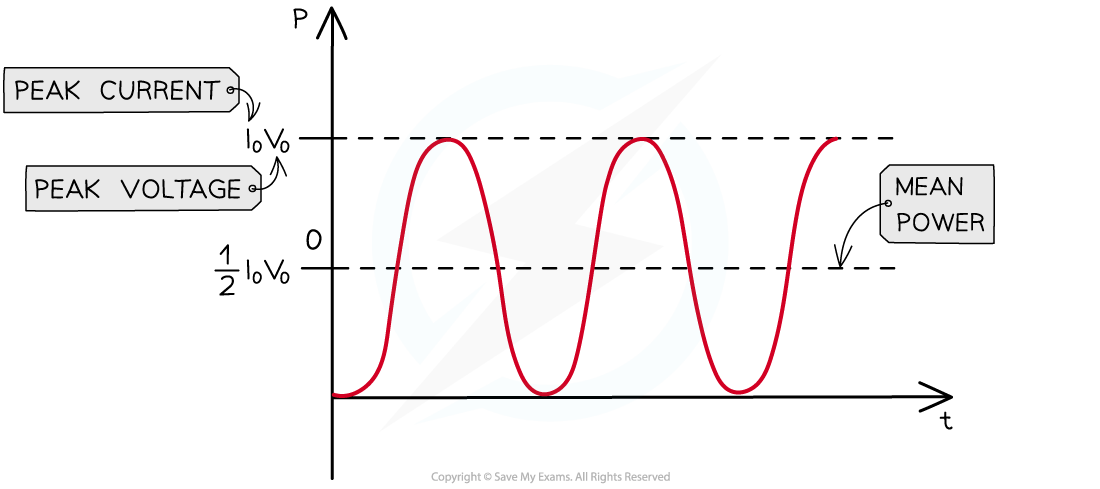# CIE A Level Physics复习笔记21.1.3 Mean Power

### Mean Power

• In mains electricity, current and voltage are varying all the time
• This also means the power varies constantly, recall the equations for power:• Where:
• I = direct   current (A)
• V = direct voltage (A)
• R = resistance (Ω)
• The r.m.s values means equations used for direct current and voltage can now be applied to alternating current  and voltage
• These are also used to determine an average current or voltage for alternating supplies
• Recall the equation for peak current:• Therefore, the peak (maximum) power is related to the mean (average) power by:
•Therefore, it can be concluded that:

The mean power in a resistive load is half the maximum power for a sinusoidal alternating current or voltageMean power is exactly half the maximum power

#### Worked Example

An alternating voltage supplied across a resistor of 40 Ω has a peak voltage V0 of 240 V.Calculate the mean power of this supply.

Step 1: Write down the known quantities

Resistance, R = 40 Ω

Peak voltage, V0 = 240 V

Step 2: Write out the equation for the peak power and calculateStep 3: Calculate the mean power

• The mean power is half of the maximum (peak) power

Mean power = 1440 / 2 = 720 W